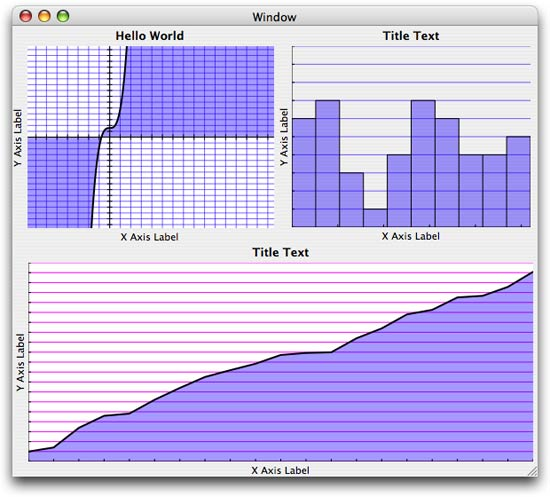The recent FaceSpan 5.0d42 build includes a graphing plugin based on Chad Weider’s GraphX framework. Chad’s framework provides three graphing views: curve, histogram and scatter plot.

As always when creating FaceSpan plugins from Cocoa frameworks, the tough part is converting the Cocoa APIs into a simple to use AppleScript syntax. In the case of these graphing views, I have substituted the ‘Data Source’ based APIs with a simple event for the curve view, and a value property that accepts a list of values to be graphed (a list of reals for the histogram and a list of x-y points for the scatter plot).

Here’s how it all looks running in a FaceSpan-built application:The code for the curve view looks like this:

```--
--  CT curve uses this event handler to get the data to be graphed
--
on CT get y value theCTCurve for theXValue
return theXValue * theXValue * theXValue + 1.0
end CT get y value
```

The event is called repeatedly by the curve view to generate the data to be graphed.

The code for the histogram looks like this:

```on initialize theResponder
--  CT histogram needs a list of reals to graph
set theData to {}

repeat with i from (x axis minimim) to (x axis maximum)
set end of theData to random number from 1 to 10
end repeat
set value to theData

end initialize
```

Here I simply generate a random series of values and assign them to the view’s value property. The plugin does the rest.

And finally, the code for the scatter plot looks like this:

```on initialize theResponder
--  CT scatter plot requires a list of points (x, y pairs) to graph...

set yValue to 1.0
set theData to {}

repeat with i from (x axis minimim) to (x axis maximum)
set end of theData to {i, yValue}
set yValue to yValue + (random number from 0 to 2.0)
end repeat
set value to theData

end initialize
```

Again, I simply generate a random series of point values and assign them to the view’s value property. The plugin does the rest.

The sources for the plugin are included in the FaceSpan 5.0d42 SDK.

1.Andre Berg
2.Mark Alldritt